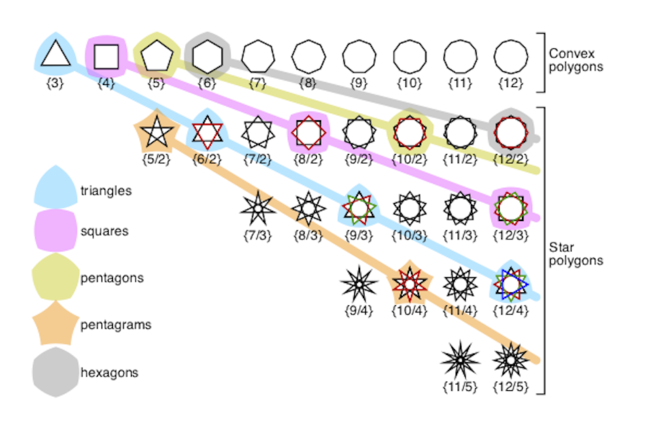## Want to keep learning?

This content is taken from the Davidson Institute of Science Education at the Weizmann Institute of Science's online course, Flexagons and the Math Behind Twisted Paper. Join the course to learn more.
3.7

## Davidson Institute of Science Education at the Weizmann Institute of Science# Les Pook’s flexagon symbols

Les Pook has written two classic books on flexagon theory. His second book, Serious Fun with Flexagons, is a rigorous, comprehensive analysis of many kinds of flexagons, the nets from which they are constructed and some of their interesting properties.

### The Schläfli symbol

Ludwig Schläfli was a 19th century Swiss mathematician who specialised in geometry, especially know for his influential work on geometry in high dimensions. Schläfli developed a symbol, the Schläfli symbol, for polygons, polyhedra and polytopes in higher dimensions. It’s quite simple. You can see the Schläfli symbols for some regular polygons in the image above. A regular polygon is a polygon whose angles are all the same and whose sides are all equal, for example an equilateral triangle, a square, a regular pentagon etc. The Schläfli symbol for a regular polygon is just the number of vertices (or edges) written inside curly brackets: {3} for an (equilateral) triangle, {4} for a square, {5} for a regular pentagon, {6} for a regular hexagon etc. The Schläfli symbol for a star polygon (a non-convex regular polygon) is a fraction written inside curly brackets. The numerator is the number of vertices and the denominator is the number of vertices skipped when drawing each edge of the star, plus 1. For example, when drawing a line from point to point on the pentagram (5-pointed star) we skips over 1 point of the star, so its Schläfli symbol is {5/2} (remember to add 1 to the denominator). The Schläfli symbol for a nonagram (9-pointed star) is {9/3} because 2 points are skipped.

### Flexagon symbols

Pook created a flexagon symbol that uses the Schläfli symbol. Pook’s flexagon symbol (usually) has 3 components. The first is the number of sectors the flexagon has. A flexagon can usually be divided into identical units. These units are called sectors. The tri-hexa-flexagon, for example, is made out of 3 identical sectors of two equilateral triangles joined at a common edge. Each sector has two pats, one of them with 1 leaf, and the other with 2 leaves. The difference in the number of leaves in each pat is the reason why there are 3 sectors and not 6. The sectors must be completely identical. The hexa-hexa-flexagon also has 3 sectors.

The second component of the flexagon symbol is the Schläfli symbol of the constituent polygon of the flexagon - the basic shape. For example, the basic constituent shape that makes both the tri-hexa- and the hexa-hexa-flexagons is a triangle. It’s Schläfli symbol is {3}, so 3 is the second component of the flexagon symbol for both these flexagons.

The third component is what’s called the flexagon net symbol. It is the Schläfli symbol of a polygon that describes the dynamics of the flexagon. This polygon, also called the associated polygon, can be constructed in the following manner. Print out the flexagon net on a transparent sheet of paper. Draw a straight line through the centre of each polygon in the flexagon net, so that it traverses the ‘hinges’ or joints between all the adjacent polygons in the net. Assemble the flexagon in its main state. The lines will form a pattern on the flexagon face. For the tri-hexa-flexagon this pattern is an equilateral triangle. Now, start pinch-flexing the flexagon, but stop when it is in an intermediate state, between main states. For the tri-hexa-flexagon this intermediate state is 3-dimensional and it looks a ‘3 pointed propeller’. Each sector is a diamond made of two triangular pats. The polygon that you will see drawn on these pats is the flexagon’s associated polygon, and its’ Schläfli symbol is the third component of the flexagon net symbol. For the tri-hexa-flexagon it is a triangle, so this component is also 3. It is very difficult to discover the associated polygon without actually making the flexagon using the procedure just described. For many of the flexagons in this course, the third component of the flexagon symbol is the same as the second component, the Schläfli symbol of the constituent polygon (don’t confuse constituent with associated) and we won’t go any further than this in this course.

Now that we know the language, we can put the pieces together and construct the flexagon symbol. It looks like this (the Schläfli squiggly brackets are omitted):

component 1<component 2, component 3>

i.e.:

Number of sectors<Schläfli symbol of the constituent polygon, Schläfli symbol of the associated polygon>

For the tri-hexa-flexagon the flexagon symbol is: 3<3,3> The magic square flexagon symbol is: 2<4,4>

### Sector symbols

Pook went one step further. He also wrote a notation for the sector of a flexagon. The notation starts off the same way as the flexagon symbol, except that the first component, the number of sectors is omitted. Two additional numbers are added to the component 2 and component 3 numbers. These are the number of leaves in each pat of the sector from the highest to the lowest. Note that for some flexagons, the sector symbol is dependent on the state of the flexagons because the sectors in different states can have a different number of leaves in each pat. The sector symbol for the tri-hexa-flexagon is <3,3,2,1>

### Discussion

This article is probably the most difficult in the course. I really hope that the explanation was clear enough and that you managed to work your way through… Let’s just see if you did… Try and answer the following questions:

1. What is the sector symbol of the magic square flexagon?
2. What is the flexagon symbol of the non-cyclic hexa-tetra-flexagon? Assume the flexagon’s associated polygon is equal to it’s constituent polygon.